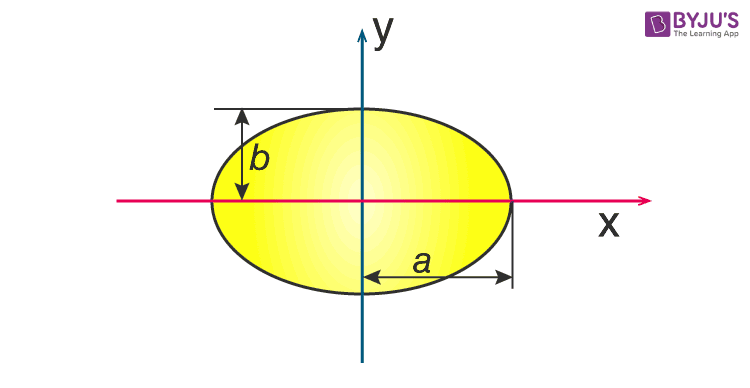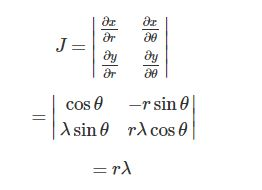Checkout JEE MAINS 2022 Question Paper Analysis : Checkout JEE MAINS 2022 Question Paper Analysis :

# Moment Of Inertia Of An Ellipse

Moment of inertia of ellipse is usually determined by the following expression;

 I = M (a2 + b2) / 4

We will further understand how this equation is derived in this article.

## Derivation of Moment of Inertia of Ellipse1. The first step involves determining the parametric equation of an ellipse.

It is given as;

(a cos t, b sin t)

Now we need to modify the parametric equation to get the transformation equation. We get;

(r cos θ, λ r sin θ)

Where

λ= b / a

Here, we have to consider a and b as the semi-major and semi-minor axis of the ellipse.

a = semi-major axis

b = semi-minor axis

We get the transformation equation as;

x = r cos θ

y = λ r sin⁡ θ

After this, we will need to find the area element using the Jacobian. It is given as;2. Finding the area

While calculating the area we have to remember that r will be integrated from 0 to semi-major axis a.

A = oa o λ r d θ

A = λ a2 π

A = π ab

3. Calculating moment of inertia

In this case, the moment of inertia formula will be;

I = ρ ∫ (x2 + y2) dA

I = ρ oa o λ r3 (cos2 θ + λ2 sin2⁡ θ) drdθ

I = ρλ a4 / 4 o (cos2 θ + λ2 sin2⁡ θ) dθ

By symmetry we get;

I = ρλ a4 ½ 2 o (cos2 θ + λ2 sin2⁡ θ) dθ

I = ρλ a4 ½ [B(½ , 3/2) + λ2b (3/2, ½ ) ]

B = beta function.

Now if we make use of the beta-gamma relation we get;

I = ρ λab / 4 (a2 + b2)

I = M (a2 + b2) / 4

Meanwhile, we also have the following equation for a filled ellipse whose radius along the x-axis is a and whose radius along the y-axis is b. It is given as;

Ix = π / 4 ab3

Iy = π / 4 a3b